# [转载]Grüneisen parameter

The Grüneisen parameter [Wiki], γth, is defined as a change in P by a change in density of E at constant V. Namely,(1)

To put it plainly, γth is the ratio of thermal pressure to thermal energy [Wiki]. Or, it describes how much P increases when the matter is heated.

It is expressed by: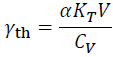(2)

If  γth is defined by this equation, it is called the thermodynamic Grüneisen parameter.

To put it plainly, what Eq (2) means is as follows.

• If E increases, the matter wants to increase its V due to α, because T should increase with E increase. Therefore, γth is proportional to α.

• If KT is higher, the potential V increase that the E increase wants to causes larger Pincrease. Accordingly, γth is proportional to KT.

• If the CV is larger, T increase becomes smaller for a given E increase, and therefore, γth is inversely proportional to CV.

In the case of MgSiO3 bridgmanite at ambient conditions, α = 2.0×10-5 K-1KT = 260×109Pa, V = 24×10-6 m3 mol-1CV is 80 J K-1 mol-1, and therefore, γth = 1.1.

Note that γth is a dimensionless parameter.

Eq. (2) is obtained from Eq. (1) by the following way.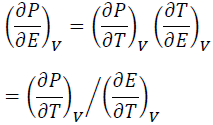(3)

The numerator of Eq (3) is:(4)

The denominator of Eq (3) is:(5)

Using Eqs (3)-(5), Eq. (2) is obtained from Eq. (1) as:(6)

The definition of the Grüneisen parameter, Eq. (1), is the Mie-Grüneisen EOS [Wiki] itself: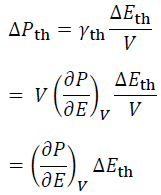(7)

There are other ways to define the Grüneisen parameter.
For example, the mode Grüneisen parameterγi, is defined as: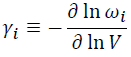(8)

where ωi is the angular frequency of the lattice vibration of the mode i.
The Debye gammaγD, is defined as: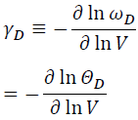(9)

where ωD is the Debye cut-off (angular) frequency [Wiki], and ΘD is the Debye temperature [Wiki].

We will discuss the mode Grüneisen parameter and Debye gamma elsewhere.

http://blog.sciencenet.cn/blog-695784-1124893.html

## 全部精选博文导读

GMT+8, 2019-12-13 05:10